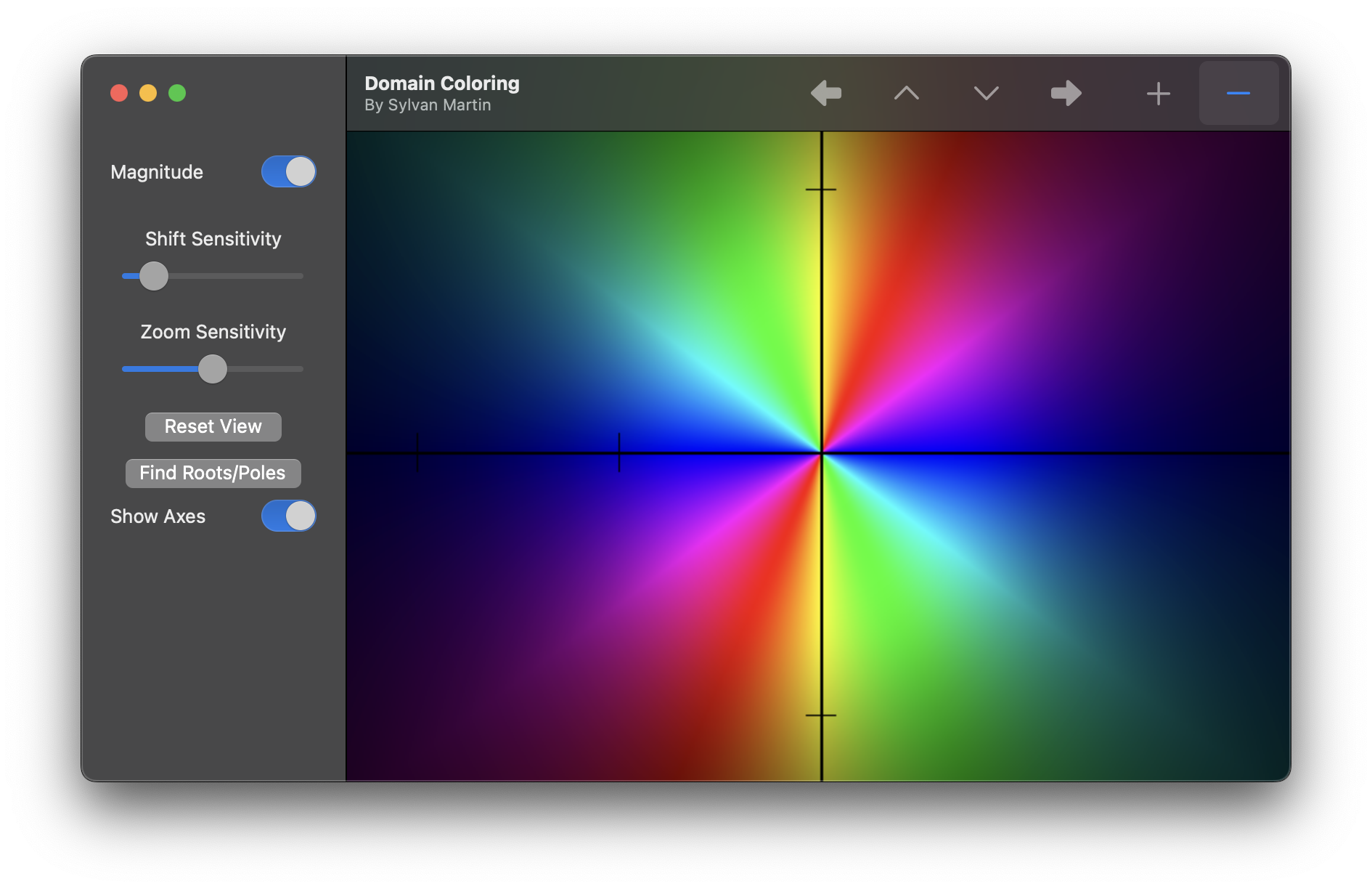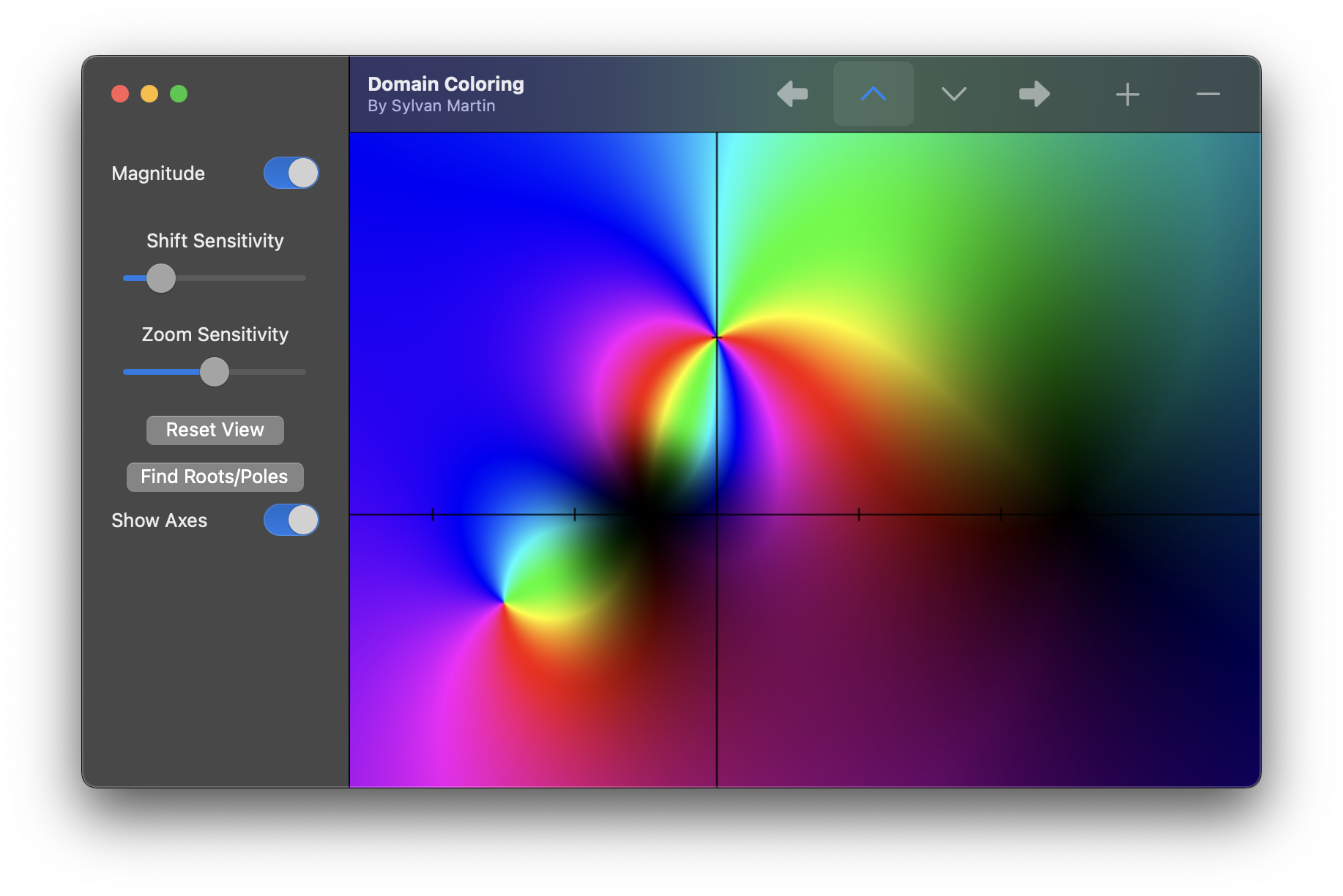# Domain-Coloring

This is a program that performs domain coloring of complex functions, as well as analyzing their properties.

## Graphing Complex Functions

This uses the same domain-coloring technique demonstrated in 3Blue1Brown’s “Winding numbers and domain coloring.”

To choose what function you want to graph, go into “Function.swift” and edit the `function(_ z: VectorType) -> VectorType` definition:

```static func function(_ z: VectorType) -> VectorType {
1 / (z * z)
}```

This will produce the graphThere are also plenty of pre-written example functions that you can play with, found in “Functions+Examples.swift” that can be used. Below is the result of using the example function `funRationalFunction`.Above is a graph of the complex rational function with a zero of order 2 at -1, a simple zero at 5, a pole of order 2 at 2i, and a simple pole at (-3 – i).

View Github